Calculus - Numerical Differentiation                                    Home : www.sharetechnote.com First Order Differentiation   < Backward Differentiation >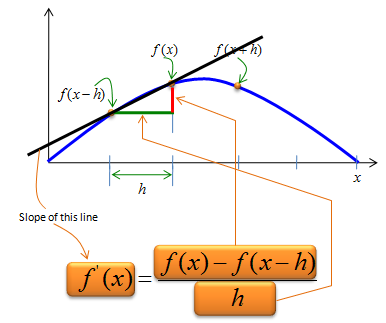< Forward Differentiation >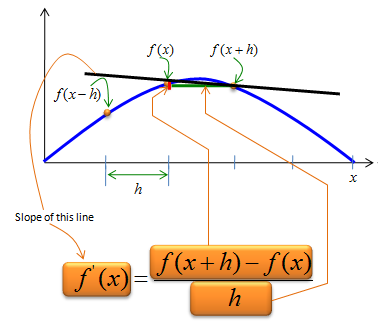< Centered Differentiation >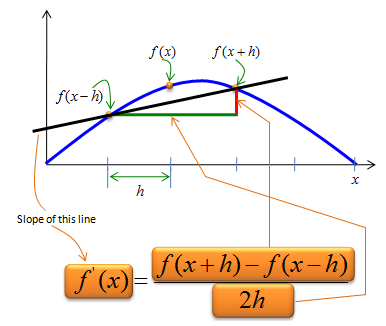Second Order Differentiation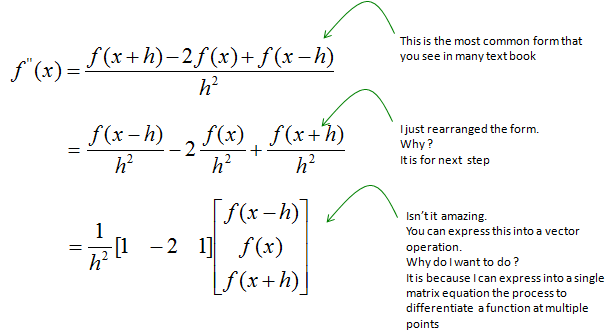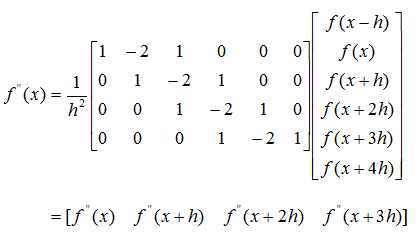< Example 1 >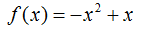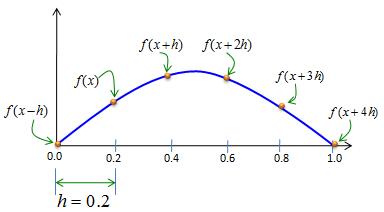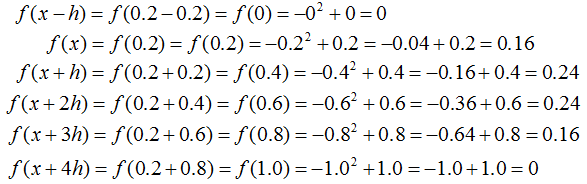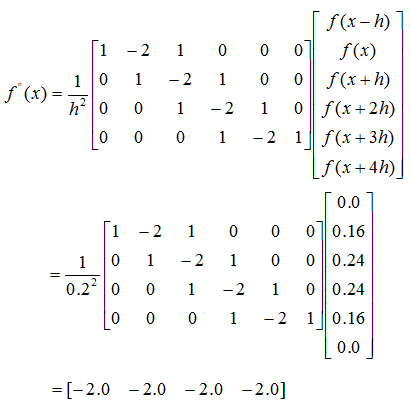If you are not confident on the calculation, click here to get the excel spreadsheet that I made.     < Example 2 >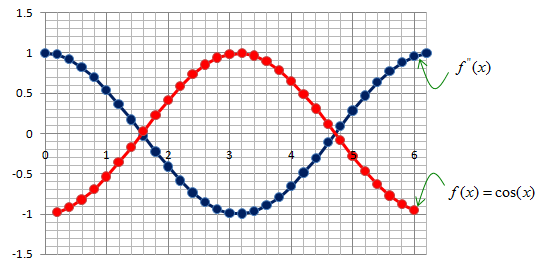Since this matrix is too large, it would be difficult to calculate manually. So I implemented this in Excel and you can get the spreadsheet here.